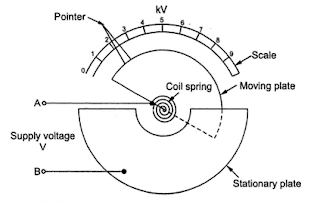### Attracted Disc Electrostatic Voltmeter

The attracted disc type instruments are generally used for the measurement of voltage above 20 Kv. The system consists of two plates such that one plate can move freely while other is fixed. Both the plates are perfectly insulated from each other. The voltage to be measured is applies across the plates as a supply voltage as shown in the Fig 1. Due to the supply voltage, electrostatic field gets produced which develops a force of attraction between the two plates. Due to the force of attraction, the movable plate gets deflected. In this mechanism the controlling torque is provided by a spring.Fig.1 Attracted Disc Type Electrostatic Voltmeter

1.1Kelving Absolute Electrometer
This instrument is of attracted disc type electrostatic voltmeter. The basic structure of this instrument is as shown in the Fig 2.Fig.2 Kelving Absolute Electrometer

The moving disc is suspended exactly above the centre of fixed form a micrometer head with the help of a spring. The moving disc is surrounded by a ring called guard ring, keeping some air gap between moving disc and ring. This guard ring is very useful in reducing fringing effects. It is connected electrically with the moving disc.
With a help of a device consisting lenses and finely pointed rod it is possible to determine zero setting of a disc consisting a fine cross-air.
In actual practice, the potential to be measured is supplied to the two disc. Due to the force attraction, the moving disc is attracted downwards. With the help of micrometer head, the moving plate is brought back to zero position setting again. This movement required is observed accurately.
For accurate measurement, it is very important to calibrate the instrument properly. During the calibration, first instrument is short circuited. Then the moving plate is set to its zero position. Then known weights are added to the disc. Then the calibration is done by observing the movements required to bring the moving disc back to the original zero position. Thus instrument measures the force of attraction produced by the potential difference between the two discs. Then the potential difference can be expressed interms of this force.
1.2 Theory of Attracted Disc Type Voltmeter
As we have seen already that the force of attraction F between the two parallel plates with potential difference V between them is given by,

Let A be the area of each plate and ε = ε0εr is the permittivity of the medium. Let d be the distance between the two plates.
Differentiating equation (2) with respect to x,
The negative sign indicates that with decrease in distance separation d, capacitance C increase. So neglecting negative sigh, the force of attraction can be rewritten as,

The potential difference V between the two plates is given by,

Thus this instrument gives an absolute determination of voltage as it is given interms of force and linear dimension. The deflecting force is adequate only when the voltage to be measured is high. For avoiding the errors due to corona effect, special construction is necessary to ensure good insulation. The superior dielectric strength of a high vacuum is used in modern instruments which enables to get more force a given voltage with very small clearance between the plates.

#### 1 comment:

1.